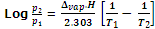Explain vapour pressure of liquid solutions.

Liquid solutions are obtained when the solvent is liquid. The solute can be a gas, liquid or a solid. In this section we will discuss the liquid solutions containing solid or liquid solutes. In such solutions the solute may or may not be volatile. We shall limit our discussion to the binary solution of the solid in liquid and liquid in liquid. Before we discuss the properties of these solution let us study about the vapour pressure of liquid.

When a liquid is taken in a beaker covered from above at certain temperature, a part of the liquid evaporates and its vapours fill the space available to them. The vapours formed will have an inclination to change back to its liquid state by the procedure of condensation. Gradually, equilibrium will be established between liquid and vapour phases. The pressure exerted by the vapours above the liquid surface in equilibrium with the liquid surface in equilibrium with the liquid at a given temperature is called vapour pressure of the liquid.

The vapour pressure of a liquid depends on Nature of liquid

Liquid which have weak intermolecular forces, are volatile and have greater vapour pressure. For instance, dimethyl ether has higher vapour pressure than ethyl alcohol.

Temperature

Vapour pressure increases with the increase in temperature. This is due to the increase in temperature through which more molecules of the liquid can go into vapour phase.

The variation of vapour pressure of a liquid with temperature is given by the Claussius Clapeyron's equation.where, p1 and p2 are vapour pressures at temperature T1 and T2 respectively. Δvap.H is enthalpy of vaporization of liquid and R is universal gas constant.

Vapour pressure of the solution of solids in liquids

Let us consider the addition of a small amount of non-volatile solute such as glucose, sucrose, sodium chloride etc. to the liquid (solvent such as water) to form a solution. In such a case the vapour pressure of the solution is solely due to the solvent, as the solute is non-volatile. It is found that the vapour pressure of the solution is lower than that of the pure solvent.

Explanation: the lowering of vapour pressure can be explained on the basis of the surface area of the liquid from which evaporation occurs. In the case of the solution, a part of the liquid surface is occupied by solute particles, which are non-volatile. Therefore, evaporation of the liquid will take place from a lesser surface area. In other words, the particles (or molecules) of the liquid will now have a less tendency to escape into vapour state. This shall, therefore, result in lowering of vapour pressure.

#### Related Questions in Chemistry

• ##### Q :Sedimentation and Velocity The first

The first method begins with a well defined layer, or boundary, of solution near the center of rotation and tracks the movement of this layer to the outside of the cell as a function of time. Such a method is termed a sedimentary velocity experiment. A

• ##### Q :What are heterogenous catalysis? Give

When the catalyst exists in a different phase than that of reactants, it is said to be heterogeneous catalyst, and the catalysis is called heterogeneous catalysis. For example, SO2 can be oxidized to SO3

• ##### Q :Cons of eating organic foods Illustrate

Illustrate the cons of eating organic foods?

• ##### Q :Question based on lowest vapour pressure

Give me answer of this question. Among the following substances the lowest vapour pressure is exerted by: (a) Water (b) Mercury (c) Kerosene (d) Rectified spirit

• ##### Q :M ive me answer of this question. When

ive me answer of this question. When mercuric iodide is added to the aqueous solution of potassium iodide, the: (a) Freezing point is raised (b) Freezing point is lowered (c) Freezing point does not change (d) Boiling point does not change

• ##### Q :Excel assignment I want it before 8 am

I want it before 8 am tomorow please. I am just wondering how much is going to be ?

• ##### Q :Problem based on lowering in vapour

Help me to solve this problem. An aqueous solution of glucose was prepared by dissolving 18 g of glucose in 90 g of water. The relative lowering in vapour pressure is: (a) 0.02 (b)1 (c) 20 (d)180

• ##### Q :Calculating Formulae Superphosphate has

Superphosphate has the formula CaH4(PO4)2 H2O, what is the calculation to get the percentage of Phosphorus, I need to show the calculation. I know it is 30.9737622 u in weight and 2 atoms of the formula, but not sure how to work the calculation backwards.

• ##### Q :Colligative properties give atleast two

give atleast two application of following colligative properties

• ##### Q :What is cannizaro reaction? Explain

Aldehydes which do not have  -hydrogen atom, such as formaldehyte and benzaldehyte, when heated with concentrated (50%)alkali solutio